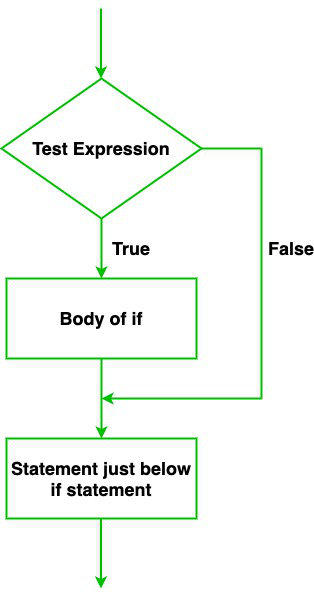# Swift – If Statement

• Last Updated : 21 Oct, 2021

Just like other programming languages in Swift language also if statement is used to execute the program based on the evaluation of one or more conditions or in other words if statement is used to run a piece of code only when the given condition is true. There are also known as branch statement. For example, let us consider a case where you’re going to go to the market, and your father tells you, “if they have the plastic container on sale, buy 2 containers”. This is a conditional statement states that you’ll perform some action, i.e., “buy 2 containers” only if the given condition, i.e., “they have the plastic container on sale” is true. Such type of statement is very useful in programming because they allow programmers to implement conditional behavior into their programs.

Syntax:

if (condition) {

// body of if statement

}

The if statement evaluates the condition inside the parenthesis() and the code inside curly braces{ } is the body of the if statement. Here,

• If the condition is fulfilled i.e. evaluated to true, then the code present in the body of the if statement is executed.
• If the condition is not fulfilled i.e. evaluated to false, the code present in the body of the if statement is skipped and the control moves to the next statement present after the if statement.

It can be better understood via visual diagram as follows :Example 1:

// Swift program of if statement

let val = 30

// Checking if number is greater than zero

if (val > 0)

{

// Code i.e. body of if statement

print(“The given number is positive.”)

}

// Statement just after if statement

// (This statement is always executed)

print(“Learning if statement in Swift.”)

Output:

```The given number is positive.
Learning if statement in Swift.```

Explanation: In the above example, we have declared a variable named “val”. Observe the test condition, “val > 0”. Here, 30 > 0 which means the condition is true so the control flow of the program enters inside the body of the if statement and executes code/statements written inside it, i.e., “The given number is positive.”. Now if we set val = -20 and run the above program then the output will be “Learning if statement in Swift”. This happens because the value of a val variable is less than 0 so the condition is false. Hence, the body of if block is skipped and the control flow of the program executes code/statements written outside/after the if statement.

Example 2:

// Swift program of if statement

let number = 30

// Checking if number is greater than equal to 18

if (number >= 18)

{

// Code i.e. body of if statement

print(“This person is eligible for voting”)

}

// Statement just after if statement

// (This statement is always executed)

print(“Only 18+ peoples are eligible for voting”)

Output :

```This person is eligible for voting
Only 18+ peoples are eligible for voting```

My Personal Notes arrow_drop_up